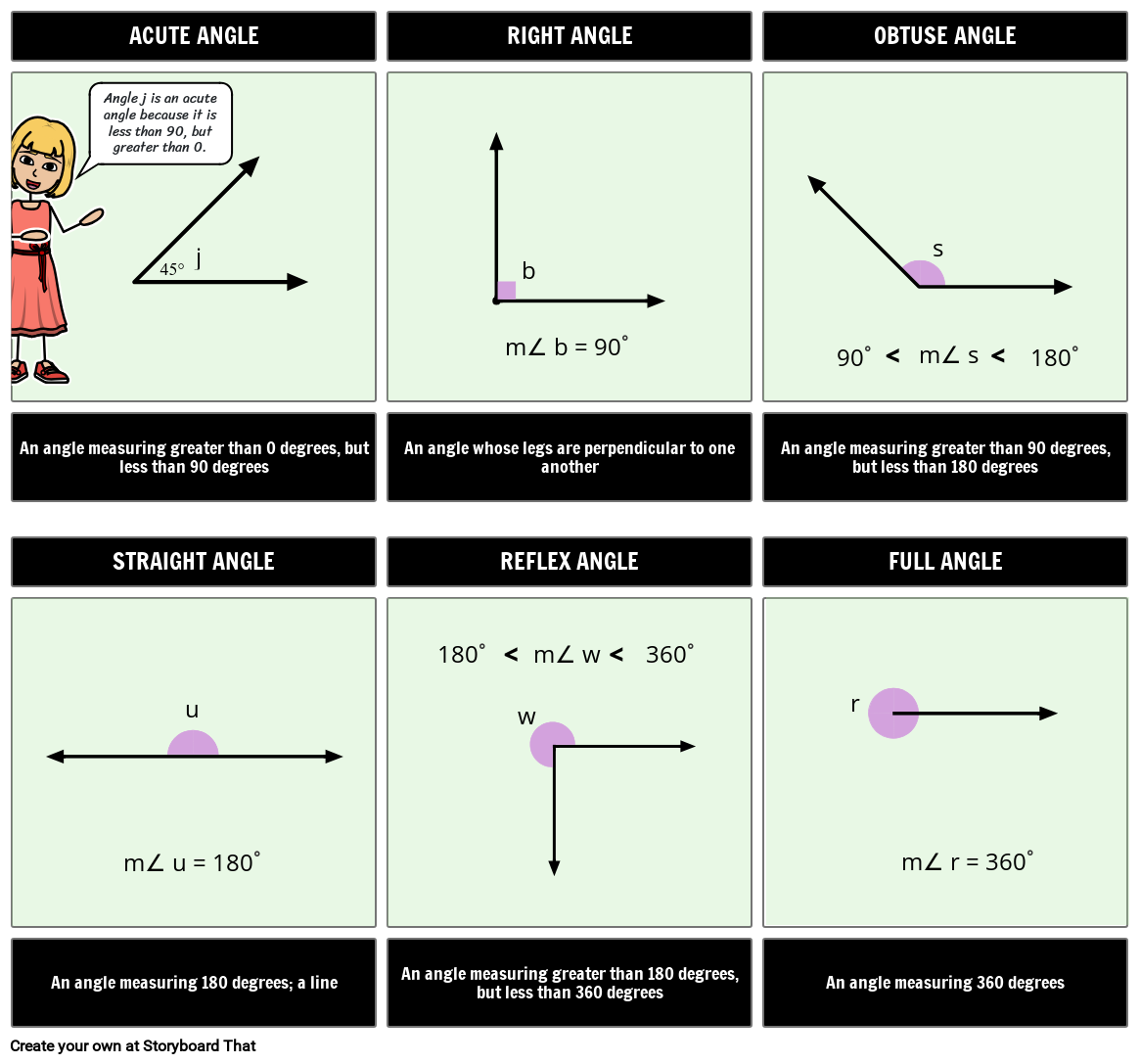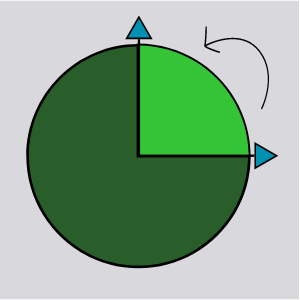# Different Types of Angles ChartYou can find this storyboard in the following articles and resources:### Introducing Angles

Lesson Plans by Anna Warfield

The basics of angles are so important for students to understand! Angles play a critical role in geometry and are the foundation for trigonometry and geometry. Students should eventually be able to read a protractor, but even just understanding if an angle is big (obtuse or reflex) or small (acute) makes a difference in elementary geometry. The following activities are a great way to provide visual aids to students and help them understand how different angles affect polygons.

### Introducing Angles

#### Storyboard Description

Create a chart describing the different types of angles.

#### Storyboard Text

• ACUTE ANGLE
• Angle j is an acute angle because it is less than 90, but greater than 0.﻿﻿﻿﻿
• ﻿j
• RIGHT ANGLE
• ﻿﻿﻿﻿﻿﻿﻿﻿﻿﻿﻿﻿﻿﻿﻿﻿﻿m∠ b = 90°
• ﻿﻿﻿b
• ﻿
• OBTUSE ANGLE
• ﻿﻿﻿﻿﻿﻿﻿90°
• ﻿﻿m∠ s
• ﻿﻿﻿﻿s
• ﻿﻿﻿﻿﻿﻿﻿﻿﻿﻿180°
• An angle measuring greater than 0 degrees, but less than 90 degrees
• STRAIGHT ANGLE
• ﻿u
• An angle whose legs are perpendicular to one another
• REFLEX ANGLE
• ﻿﻿﻿﻿﻿﻿﻿﻿﻿﻿﻿﻿﻿180°
• ﻿﻿﻿﻿﻿﻿﻿﻿﻿﻿﻿﻿m∠ w
• ﻿﻿﻿﻿﻿﻿﻿﻿﻿﻿﻿﻿﻿﻿﻿360°
• An angle measuring greater than 90 degrees, but less than 180 degrees
• FULL ANGLE
• ﻿r
• An angle measuring 180 degrees; a line
• ﻿﻿﻿﻿﻿﻿﻿﻿﻿﻿﻿﻿﻿﻿﻿﻿﻿﻿﻿﻿m∠ u = 180°
• An angle measuring greater than 180 degrees, but less than 360 degrees
• ﻿w
• An angle measuring 360 degrees
• ﻿﻿﻿﻿﻿﻿﻿﻿﻿﻿﻿﻿﻿﻿﻿﻿﻿﻿﻿﻿﻿m∠ r = 360°
##### Over 20 Million Storyboards Created